## Monday, June 18, 2012

### The Fifth Degree: Part II

When we left off, I said there was a problem with the solution of the cubic equation. Any formula powerful enough to do the job would generate too many solutions. Once you take the square root of this and the cube root of that, you already have six values. How do you write a formula that only generates three values, as required?

The solution to this problem is beautiful and unexpected. First let's look again at the hypothetical form I had for a solution of the cubic: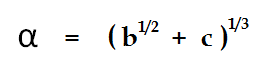This is actually a slight simplification. You may remember that I had three terms: a,b, and c in my earlier formula. I've thrown out the "a" term because it represents a simple linear shift that's easy to get rid of by simple algebra no matter what the degree of your equation. The formula I'm showing here is the basic form, and like I said, it generates six values instead of the required three.

Here's the funny thing: we can list all six values, and it turns out they can be combined with each other in a particular way that reduces them to just three values. We start by noticing that there are two groups of values for the cube roots, depending on our choice of the square root. For alternate values of the square root of b, we can call our cube roots p and q: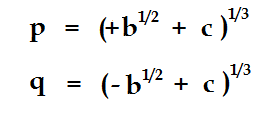You shouldn't think of the positive and negative square roots of b as separate and identifiable numbers. They are indeed two different numbers, but there is at the same time something indistinguishable about them. If b is a negative number, then they represent alternate choices for the imaginary i. It should be obvious that the sign of i is a simple matter of human convention, and that mathematically speaking there is no way to distinguish the "positive" and "negative" square roots of minus one. What is not so obvious to most people is that in terms of algebra, the positive and negative square roots of ordinary numbers like 2 are just as indistinguishable! At first thought, this is crazy talk...the number 1.414... is clearly a different number from the number -1.414...: one is positive and one is negative. This is not the right way to think about it! In algebra, a number is defined strictly by its algebraic properties with respect to the rational numbers. All we can say about the number we call "the square root of two" is that when you square it, you get the rational number 2. It is simply outside the scope of the discussion to say that one is positive and the other is negative.

The same goes for the cube roots! We know there are three cube roots of two: the real one, and the two complex ones located 120 degrees apart on the circle passing through the real root. Here is the strange thing about algebra: there is no meaningful distinction between those three roots! You can't tell them apart algebraically. What's more, it does you no good to try and tell them apart.

Of course, this doesn't apply to the cube roots of eight. The real cube root is 2, a rational number, and the complex roots are different numbers altogether. The two complex roots are indistinguishable from each other, but the rational root is special.

What about our p's and q's....where p and q are distinguished by the choice of the square root? Here it gets a little more interesting. Let's start by listing the six values for the expression. Letting omega be a complex cube root of unity (it could be either one, it doesn't matter!) we get these six terms: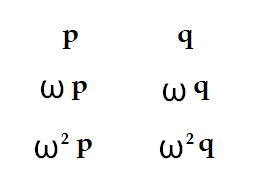I pointed out last time that these six terms are actually the roots of a sixth-degree equation. Now comes the magical part. By mixing and matching, I can construct the roots of a cubic equation. First I'm going to tell you what they look like, and then we'll talk about why it works. First, here is what the roots look like: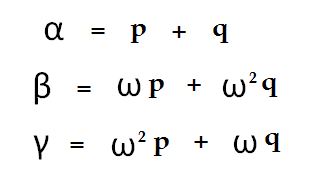We've taken six expressions and collapsed them down to three expressions. Two choices for the square roots and three choices for the cube root, and yet in the end we get three unique values.

Or do we? Haven't I cheated? Earlier I claimed that the three cube roots of a number are algebraically indistinguishable from each other. If they really are, then how am I entitled put the three cube roots in a specific order? In particular, I choose "p+q" as my alpha, from which beta and gamma follow. What would have stopped me from choosing , for example: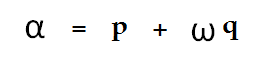as my first root....then I would have gotten entirely differnt values for beta and gamma as well.  For that matter, I might have chosen: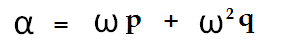but that wouldn't have hurt me, because while I would have gotten correspondingly different values for alpha, beta and gamma, they would have been the same three values that I got the first time around....just their names would have been swapped. But the other choice....where I take p+wq....that gives me three different values altogether. If I really can't distinguish between the three cube roots of a number, how do I choose which cube roots line up with each other? Do I have to take recourse to Cartesian geometry and recognize the "real" cube root as being distinguished from the others? Or is there some purely algebraic property which lets me identify three unique values for the roots of the equation?

It's a funny question and I think I'll leave the answer for tomorrow.